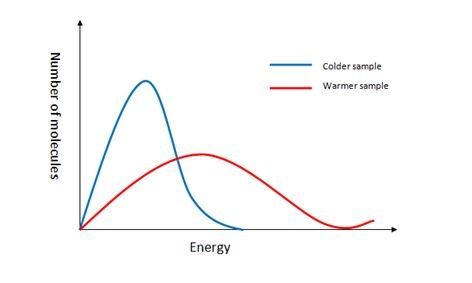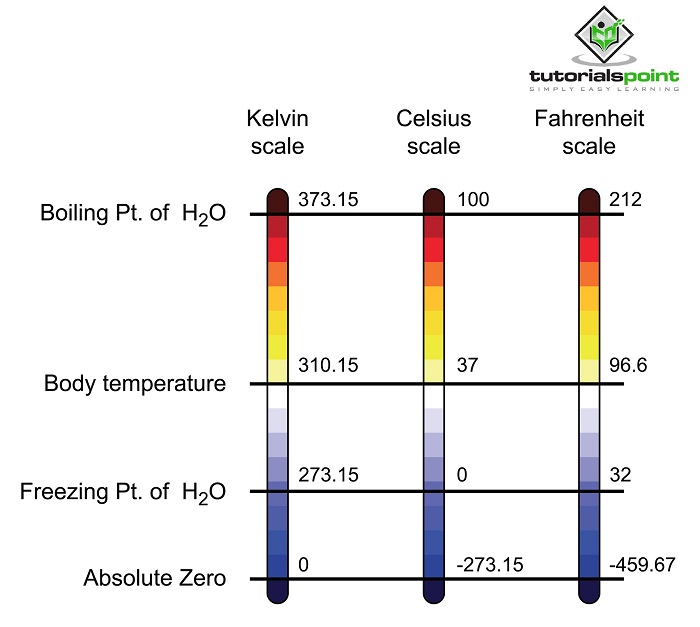# Temperature

## Introduction

Temperature is an -important aspect of science and it is the most important aspect of daily life. It is seen as the measurement of the average kinetic energy of the articles of an object. It is seen that with the increase in temperature, particle motions also increase. It is seen when the particles move first, and then the temperature increases more.

## Definition of Temperature

It is seen that temperature is the expression through which the coldness or hotness of a body can be defined. The expression is done through different scales like Celsius and Fahrenheit. Temperature is nothing but an indication of the direction in which the energy of heat flows continuously (Adeeb, Farhan & Al-Salaymeh, 2019). Heat flows from a hotter body to a colder body. It is the most important part that temperature defines the direction of heat flow keeping bodies at different temperatures in contact. It is expressed through the SI unit Kelvin (K).

## Relationship between Temperature and Kinetic Energy

There is a relation between Temperature and Kinetic Energy and it says that a substance’s temperature is directly proportional to the substance particle’s average kinetic energy. In this field, the mass of the particles is constant and that is why the particles move faster at the time of rising temperature.It is seen that there is an effect of the increase in temperature within kinetic energy. When the temperature of a system is lowered, then the kinetic energy of the particle also decreases.

## Difference between Temperature and Heat

Heat and Temperature are two different concepts, although they are studied together in science. The concept of Heat says that it is a form of energy but temperature, on the other hand, is a measure of energy. Heat is considered to be the overall energy of the motion of a molecule but the temperature is the average energy of the molecular motion. Heat can be said that as an amount of energy in the body but temperature means the measurement of the intensity of heat. Heat flows from the hotter object to the cooler object. The temperature rises when it is heated, but falls when it cools.

Images Coming soon

## Measurement of temperature

The measurement of temperature is done using a Thermometer. It is seen that the thermometer is the specific instrument for the measurement of temperature. Liquid thermometer is the simplest one, they are thin glass tubes, and it is filled with mercury (Adeeb, Farhan & Al-Salaymeh, 2019).

Thermal expansion helps in the measurement of temperature to the Thermometers. In the time of measuring temperature, it is seen that change in the temperature is simply the change in the volume of the liquid. The mercury gets high when the temperature is increased and it is the most common process for measuring the temperature.

## Temperature sensor

Temperature sensor is described as a Resistance Temperature Detector or it can also be called a thermocouple. It helps to collect data from a particular source and converts it into an understandable unit. A thermometer here is an example of a temperature sensor and it helps to measure the temperature of gases, liquids, and solids. There are some other sensors of temperature such as Semiconductors, Infrared sensors, Thermocouples, Thermistors, and Resistor temperature detectors.

## Scales of Temperature

It is seen that Thermometers helps to measure temperature and there are specific scales for measurement. Three common types of scales are- Kelvin, Celsius, and Fahrenheit. Kelvin scale is one of the most common scales and it is an absolute temperature scale and the lowest temperature is defined as 0K. The boiling point of water is 373.15 K. Fahrenheit scale contains the freezing point of water is 32ºF and the boiling point is 212ºF. Celsius scale ha the boiling point of water is 100ºC and the cooling point is 0ºC.## Concept of Thermal Equilibrium

Thermal Equilibrium is the concept which is established when two bodies met each other. In this situation, they have the ability to freely exchange energy. The most important factor here is that thermometer does not take the temperature of another object; rather it takes its own temperature (Sun et al. 2022). It is seen that the heat of an object flows from the hotter one to the cooler one until both of them reach the same temperature. These objects are called the Thermal Equilibrium.

## Conclusion

Temperature mainly defines the hotness and coldness of a body. It is seen that when the movement of the particles becomes faster than temperature also increases and with the slowdown of the particles, temperature decreases. A thermometer helps in the measurement of temperature and it has three types of scales for measurement. It is seen that there is a proportional relationship between Temperature and Kinetic Energy.

## FAQs

Q.1. What is the definition of temperature?

Ans. Temperature means the average kinetic energy of all the molecules together. It can also say as the average energy of all the particles within an object.

Q.2. What is the definition of heat?

Ans. Heat in a particular object describes the energy of all molecular movement inside an object. It is a kind of energy and it can transform from one object to another object.

Q.3. Is there any difference between heat and temperature?

Ans. There is a key difference between these two matters. Temperature is the measurement of the intensity of heat whereas heat is the amount of energy in a body.

Q.4. What are the names of scales for a temperature measurement?

Ans. There are three common types of scales for the measurement of temperature. They are- Fahrenheit (F), Celsius (C), and Kelvin (K). These are the units for the measurement of temperature.